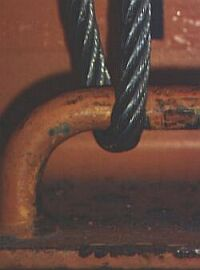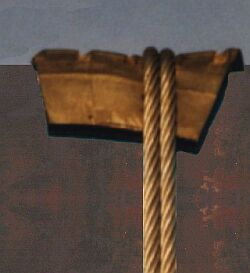5.2.14.17   Slabs - maximum securing load of wire ropesSteel wire rope lashing is another option, for example wire ropes of the type 6 x 37 + 1 FC in conjunction with suitable shackles and turnbuckles would be a usable alternative.

The "Guidelines for the correct stowage and securing of cargoes for carriage in ocean-going ships" provide the following rule of thumb for calculating the nominal breaking load (BL) of steel wire ropes of the stated type: BL = 50 x d².

The basic calculations for 10 mm thick wire ropes are as follows:

 BL = d x d x 50 = 1 x 1 x 50 = 50 [kN] BL = d x d x 50 = 10 x 10 x 50 = 5,000 [daN]

The basic calculations for 12 mm thick wire ropes are as follows:

 BL = d x d x 50 = 1.2 x 1.2 x 50 = 72 [kN] BL = d x d x 50 = 12 x 12 x 50 = 7,200 [daN]

Since the regulations state that the maximum securing load for "single-use wire ropes" can be assumed to be only 80% of the breaking load, the MSL of the above-described lashing wire ropes can be calculated as follows:

For 10 mm diameter wire ropes:

 MSL = BL x 0.8 = 50 x 0.8 = 40 [kN] MSL = BL x 0.8 = 5,000 x 0.8 = 4,000 [daN]

For 12 mm diameter wire ropes:

 MSL = BL x 0.8 = 72 x 0.8 = 57.6 [kN] MSL = BL x 0.8 = 7,200 x 0.8 = 5,760 [daN]

A direct rule of thumb can be used to calculate the MSL of "single-use wire lashing ropes" from the above-stated values without having to calculate the BL. Since the MSL can amount to at most 80% of the BL, the rule of thumb for the MSL of "single-use wire lashing ropes" is:

 MSL = d² x 40

The direct calculation using the rule of thumb for 10 mm wire ropes would thus have been:

 MSL = d x d x 40 = 1 x 1 x 40 = 40 [kN] MSL = d x d x 40 = 10 x 10 x 40 = 4,000 [daN]

The maximum securing load of 12 mm wire ropes could have been calculated as follows:

 MSL = d x d x 40 = 1.2 x 1.2 x 40 = 57.6 [kN] MSL = d x d x 40 = 12 x 12 x 40 = 5,760 [daN]

The calculated strengths apply per single run of the particular rope and should be multiplied appropriately for multiple runs of the rope. A note of caution must be sounded, however, as wire ropes are weakened by deflection around lashing points, shackles, turnbuckles or other fastening elements, the extent of which weakening being greater, the smaller is the ratio of diameter of deflection D to rope diameter d.

 D/d ratio 0.5 1.0 1.5 2.0 2.5 3.0 3.5 4.0 4.5 5.0 Strength per single run 50% 65% 72% 77% 81% 85% 89% 93% 96% 99%Example of weakening of a wire rope of 16 mm diam. on deflection around a lashing point of 20 mm diam. and example of nonuniform securing

The lashing point has a diameter of D = 20 mm, the wire rope of d = 16 mm.

The D/d ratio is 20 mm / 16 mm = 1.25.

On the basis of the Table, strength per single run can be calculated as approx. 68% that of the single run.

On the basis of the above-stated rules of thumb, the theoretical nominal breaking load of the doubled, 1.6 cm or 16 mm diam. wire rope is thus

1.6 x 1.6 x 50 x 0.68 = 87.04 kN or
d² x 50 x 0.68 = 16 x 16 x 50 x 0.68 = 8,704 daN

Since the MSL of "single-use wire ropes" can amount to at most 80% of the BL, the maximum securing load of the doubled wire rope is

87.04 kN x 0.8 = 69 kN or
8,704 daN x 0.8 = 6,963.2 daN.

The MSL of the 20 mm diam. lashing point is 50% of the BL, i.e. d² x 20 x 0.5.
Depending upon whether the result is required in daN or kN, the calculations are as follows:

 MSL = d² x 20 x 0.5 = 2 x 2 x 20 x 0.5 = 40 [kN] MSL = d² x 20 x 0.5 = 20 x 20 x 20 x 0.5 = 4,000 [daN]

Since the doubled wire rope has a substantially greater loading capacity than the lashing point to which it is attached, material was wasted in this case. Applying 16 mm wire rope securing to a 20 mm lashing point is nonuniform and uneconomic.

However, as the following Table shows, this does not only apply to the 16 mm wire rope. With the 20 mm diameter lashing point in question, it is only economic to use the 8 mm diameter "single-use wire lashing ropes", because in this case the maximum securing load of the double run virtually matches the maximum securing load of the lashing point. Smaller diameters of rope do not fully utilize the strength of the lashing point, while larger diameters do not fully utilize the strength of the wire lashing ropes.

 Ropediam-eter[mm] Breaking load of a double run Percent-age MSL of BL Ratio of lashing point & wire diam. Residual strength after deflection MSL of double run taking account of deflection d 2 ū d² ū 50 80% D/d as per Table Rule of thumb [in kN] 6 2 ū 0.6 ū 0.6 ū 50 0.8 20/6 = 3.33 approx. 87% 25.056 8 2 ū 0.8 ū 0.8 ū 50 0.8 20/8 = 2.50 81% 41.472 10 2 ū 1.0 ū 1.0 ū 50 0.8 20/10 = 2.00 77% 61.600 12 2 ū 1.2 ū 1.2 ū 50 0.8 20/12 = 1.66 approx. 74% 85.248 14 2 ū 1.4 ū 1.4 ū 50 0.8 20/14 = 1.43 approx. 71% 111.328 16 2 ū 1.6 ū 1.6 ū 50 0.8 20/16 = 1.25 approx. 68% 139.264

In the case of reusable wire lashing ropes, the "Guidelines for the correct stowage and securing of cargoes for carriage in ocean-going ships" state that the maximum securing load may be deemed to be only 30% of the nominal breaking load.

The following Table states MSL values for various thicknesses and deflections of such wires:

 Ropediam-eter[mm] Breaking load of a double run Percent-age MSL of BL Ratio of lashing point & wire diam. Residual strength after deflection MSL of double run taking account of deflection d 2 ū d² ū 50 30% D/d as per Table Rule of thumb [in kN] 6 2 ū 0.6 ū 0.6 ū 50 0.3 20/6 = 3.33 approx. 87% 9,396 8 2 ū 0.8 ū 0.8 ū 50 0.3 20/8 = 2.50 81% 15.552 10 2 ū 1.0 ū 1.0 ū 50 0.3 20/10 = 2.00 77% 23.100 12 2 ū 1.2 ū 1.2 ū 50 0.3 20/12 = 1.66 approx. 74% 31.968 14 2 ū 1.4 ū 1.4 ū 50 0.3 20/14 = 1.43 approx. 71% 41.748 16 2 ū 1.6 ū 1.6 ū 50 0.8 20/16 = 1.25 approx. 68% 52.224

Clearly, according to this Table, only the use of 14 mm diameter "multiple-use wire ropes" makes sense.

"Sharp edges" bring about a particularly marked reduction in the strength of steel wire ropes. Appropriate protective measures are thus very highly advisable.Edge protection for wire ropes on a slab provided by a tire segment

Wire ropes can be applied in various different ways. These various methods require the use of different strengths/thicknesses of materials and different kinds of aids.Contact  |  Site Map  |  Glossary  |  Legal Notice  |  Paper version